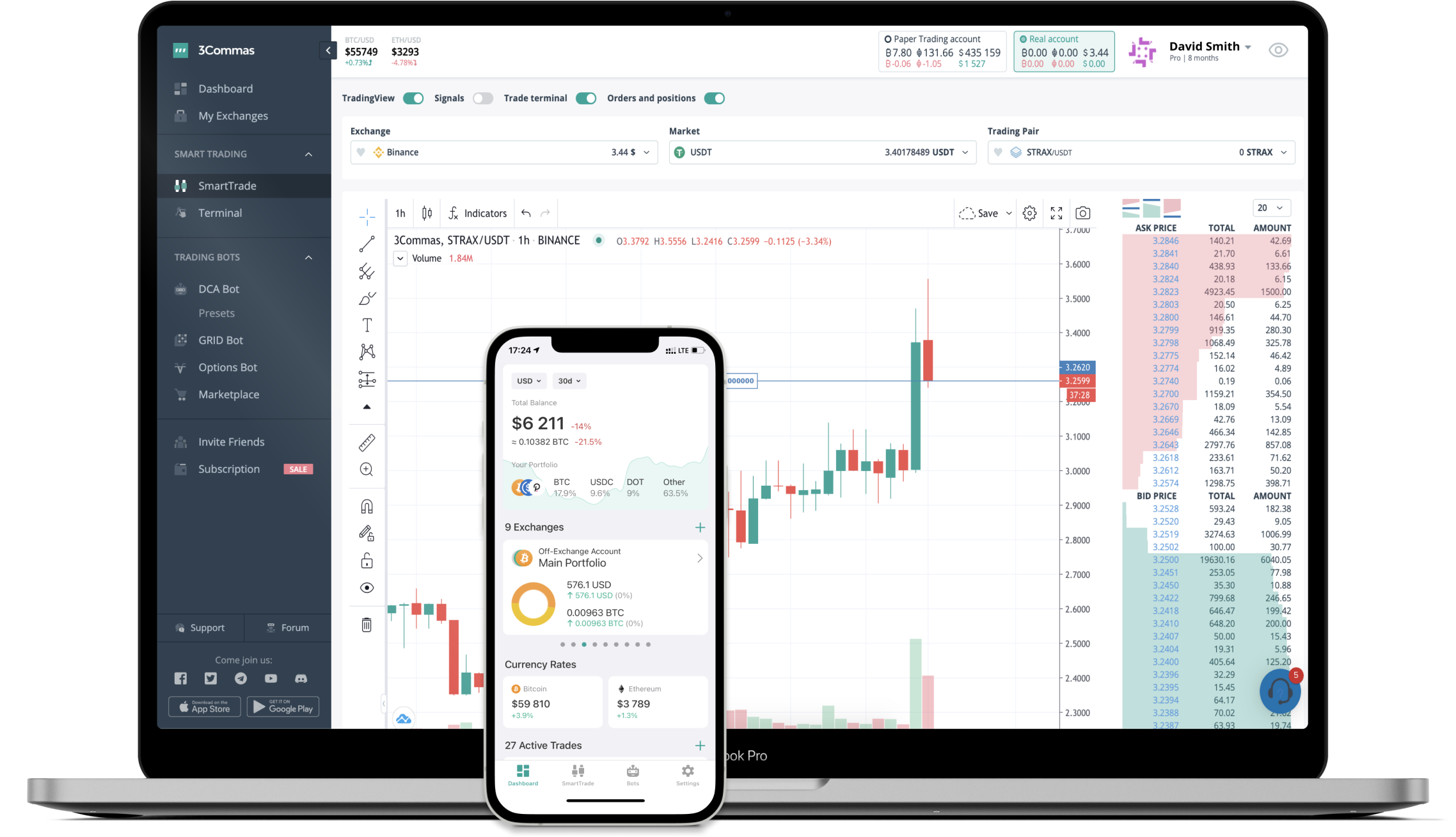# EQUAL to GBP Converter

Equalizer DEX
PriceGBP 5.82-5.38%
VolumeGBP 223,262
How it work

The 3Commas currency calculator allows you to convert a currency from Equalizer DEX (EQUAL) to British Pound (GBP) in just a few clicks at live exchange rates.

Simply enter the amount of Equalizer DEX you wish to convert to GBP and the conversion amount automatically populates. You can also use our Prices Calculator Table to calculate how much your currency is worth in other denominations, i.e. .1 EQUAL, .5 EQUAL, 1 EQUAL, 5 EQUAL, or even 10 EQUAL.

## EQUAL to GBP Exchange Calculator

The chart displays Equalizer DEX price changes in GBP.

The ultimate tools for crypto traders to maximize trading profits while minimizing risk and loss.## Latest EQUAL to GBP price calculator

Equalizer DEX market price is updated every three minutes and is automatically displayed in GBP. Below are the most popular denominations to convert to GBP.

Equalizer DEX
British Pound
0.06
GBP
0.58
GBP
5.82
GBP
11.64
GBP
17.46
GBP
29.10
GBP
58.20
GBP
145.50
GBP
291.00
GBP
582.00
GBP
1455.00
GBP
2910.00
GBP
5820.00
GBP
14550.00
GBP
British Pound
Equalizer DEX
0.00171821
EQUAL
0.01718213
EQUAL
0.17182131
EQUAL
0.34364261
EQUAL
0.51546392
EQUAL
0.85910653
EQUAL
1.71821306
EQUAL
4.29553265
EQUAL
8.59106529
EQUAL
17.18213058
EQUAL
42.95532646
EQUAL
85.91065292
EQUAL
171.82130584
EQUAL
429.55326460
EQUAL

## Top Currencies